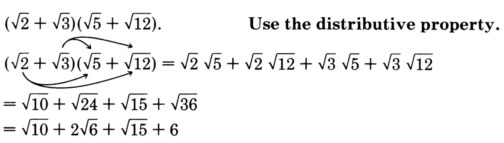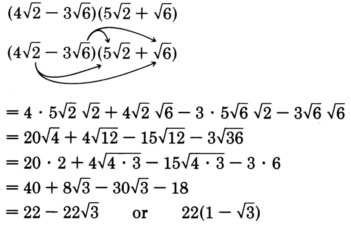# 9.5 Addition and subtraction of square root expressions

 Page 1 / 1
This module is from Elementary Algebra by Denny Burzynski and Wade Ellis, Jr. The distinction between the principal square root of the number x and the secondary square root of the number x is made by explanation and by example. The simplification of the radical expressions that both involve and do not involve fractions is shown in many detailed examples; this is followed by an explanation of how and why radicals are eliminated from the denominator of a radical expression. Real-life applications of radical equations have been included, such as problems involving daily output, daily sales, electronic resonance frequency, and kinetic energy.Objectives of this module: understand the process used in adding and subtracting square roots, be able to add and subtract square roots.

## Overview

• The Logic Behind The Process
• The Process

## The logic behind the process

Now we will study methods of simplifying radical expressions such as

$\begin{array}{ccccc}4\sqrt{3}+8\sqrt{3}& & \text{or}& & 5\sqrt{2x}-11\sqrt{2x}+4\left(\sqrt{2x}+1\right)\end{array}$

The procedure for adding and subtracting square root expressions will become apparent if we think back to the procedure we used for simplifying polynomial expressions such as

$\begin{array}{ccccc}4x+8x& & \text{or}& & 5a-11a+4\left(a+1\right)\end{array}$

The variables $x$ and $a$ are letters representing some unknown quantities (perhaps $x$ represents $\sqrt{3}$ and $a$ represents $\sqrt{2x}$ ). Combining like terms gives us

$\begin{array}{ccccc}4x+8x=12x\hfill & \hfill & \text{or}\hfill & \hfill & 4\sqrt{3}+8\sqrt{3}=12\sqrt{3}\hfill \\ \text{and}\hfill & \hfill & \hfill & \hfill & \hfill \\ 5a-11a+4\left(a+1\right)\hfill & \hfill & \text{or}\hfill & \hfill & 5\sqrt{2x}-11\sqrt{2x}+4\left(\sqrt{2x}+1\right)\hfill \\ 5a-11a+4a+4\hfill & \hfill & \hfill & \hfill & 5\sqrt{2x}-11\sqrt{2x}+4\sqrt{2x}+4\hfill \\ -2a+4\hfill & \hfill & \hfill & \hfill & -2\sqrt{2x}+4\hfill \end{array}$

## The process

Let’s consider the expression $4\sqrt{3}+8\sqrt{3}.$ There are two ways to look at the simplification process:

1. We are asking, “How many square roots of 3 do we have?”

$4\sqrt{3}$ means we have 4 “square roots of 3”

$8\sqrt{3}$ means we have 8 “square roots of 3”

Thus, altogether we have 12 “square roots of 3.”
2. We can also use the idea of combining like terms. If we recall, the process of combining like terms is based on the distributive property

$\begin{array}{ccccc}4x+8x=12x& & \text{because}& & 4x+8x=\left(4+8\right)x=12x\end{array}$

We could simplify $4\sqrt{3}+8\sqrt{3}$ using the distributive property.

$4\sqrt{3}+8\sqrt{3}=\left(4+8\right)\sqrt{3}=12\sqrt{3}$

Both methods will give us the same result. The first method is probably a bit quicker, but keep in mind, however, that the process works because it is based on one of the basic rules of algebra, the distributive property of real numbers.

## Sample set a

$-6\sqrt{10}+11\sqrt{10}=5\sqrt{10}$

$\begin{array}{ccccc}4\sqrt{32}+5\sqrt{2}.\hfill & \hfill & \hfill & \hfill & \text{Simplify}\text{}\sqrt{32}.\hfill \\ 4\sqrt{16\text{}·\text{}2}+5\sqrt{2}\hfill & =\hfill & 4\sqrt{16}\sqrt{2}+5\sqrt{2}\hfill & \hfill & \hfill \\ \hfill & =\hfill & 4\text{}·\text{}4\sqrt{2}+5\sqrt{2}\hfill & \hfill & \hfill \\ \hfill & =\hfill & 16\sqrt{2}+5\sqrt{2}\hfill & \hfill & \hfill \\ \hfill & =\hfill & 21\sqrt{2}\hfill & \hfill & \hfill \end{array}$

$\begin{array}{ccccc}-3x\sqrt{75}+2x\sqrt{48}-x\sqrt{27}.\hfill & \hfill & \hfill & \hfill & \text{Simple each of the three radicals}\text{.}\hfill \\ \hfill & =\hfill & -3x\sqrt{25\text{}·\text{}3}+2x\sqrt{16\text{}·\text{}3}-x\sqrt{9\text{}·\text{}3}\hfill & \hfill & \hfill \\ \hfill & =\hfill & -15x\sqrt{3}+8x\sqrt{3}-3x\sqrt{3}\hfill & \hfill & \hfill \\ \hfill & =\hfill & \left(-15x+8x-3x\right)\sqrt{3}\hfill & \hfill & \hfill \\ \hfill & =\hfill & -10x\sqrt{3}\hfill & \hfill & \hfill \end{array}$

$\begin{array}{ccccc}5a\sqrt{24{a}^{3}}-7\sqrt{54{a}^{5}}+{a}^{2}\sqrt{6a}+6a.\hfill & \hfill & \hfill & \hfill & \text{Simplify each radical}\text{.}\hfill \\ \hfill & =\hfill & 5a\sqrt{4\text{}·\text{}6\text{}·\text{}{a}^{2}\text{}·\text{}a}-7\sqrt{9\text{}·\text{}6\text{}·\text{}{a}^{4}\text{}·\text{}a}+{a}^{2}\sqrt{6a}+6a\hfill & \hfill & \hfill \\ \hfill & =\hfill & 10{a}^{2}\sqrt{6a}-21{a}^{2}\sqrt{6a}+{a}^{2}\sqrt{6a}+6a\hfill & \hfill & \hfill \\ \hfill & =\hfill & \left(10{a}^{2}-21{a}^{2}+{a}^{2}\right)\sqrt{6a}+6a\hfill & \hfill & \hfill \\ \hfill & =\hfill & -10{a}^{2}\sqrt{6a}+6a\hfill & \hfill & \begin{array}{l}\text{Factor out}-2a\text{.}\hfill \\ \text{(This step is optional}\text{.)}\hfill \end{array}\hfill \\ \hfill & =\hfill & -2a\left(5a\sqrt{6a}-3\right)\hfill & \hfill & \hfill \end{array}$

## Practice set a

Find each sum or difference.

$4\sqrt{18}-5\sqrt{8}$

$2\sqrt{2}$

$6x\sqrt{48}+8x\sqrt{75}$

$64x\sqrt{3}$

$-7\sqrt{84x}-12\sqrt{189x}+2\sqrt{21x}$

$-48\sqrt{21x}$

$9\sqrt{6}-8\sqrt{6}+3$

$\sqrt{6}+3$

$\sqrt{{a}^{3}}+4a\sqrt{a}$

$5a\sqrt{a}$

$4x\sqrt{54{x}^{3}}+\sqrt{36{x}^{2}}+3\sqrt{24{x}^{5}}-3x$

$18{x}^{2}\sqrt{6x}+3x$

## Sample set b$\begin{array}{ccccc}\frac{3+\sqrt{8}}{3-\sqrt{8}}.& & & & \begin{array}{l}\text{We'll\hspace{0.17em}rationalize\hspace{0.17em}the\hspace{0.17em}denominator\hspace{0.17em}by\hspace{0.17em}multiplying\hspace{0.17em}this\hspace{0.17em}fraction}\\ \text{by\hspace{0.17em}1\hspace{0.17em}in\hspace{0.17em}the\hspace{0.17em}form}\frac{3+\sqrt{8}}{3+\sqrt{8}}.\end{array}\\ \frac{3+\sqrt{8}}{3-\sqrt{8}}\text{\hspace{0.17em}}·\text{\hspace{0.17em}}\frac{3+\sqrt{8}}{3+\sqrt{8}}& =& \frac{\left(3+\sqrt{8}\right)\left(3+\sqrt{8}\right)}{{3}^{2}-{\left(\sqrt{8}\right)}^{2}}& & \\ & =& \frac{9+3\sqrt{8}+3\sqrt{8}+\sqrt{8}\sqrt{8}}{9-8}& & \\ & =& \frac{9+6\sqrt{8}+8}{1}& & \\ & =& 17+6\sqrt{8}& & \\ & =& 17+6\sqrt{4\text{\hspace{0.17em}}·\text{\hspace{0.17em}}2}& & \\ & =& 17+12\sqrt{2}& & \end{array}$

$\begin{array}{ccccc}\frac{2+\sqrt{7}}{4-\sqrt{3}}.\hfill & \hfill & \hfill & \hfill & \begin{array}{l}\text{Rationalize the denominator by multiplying this fraction by}\hfill \\ \text{1 in the from}\frac{4+\sqrt{3}}{4+\sqrt{3}}.\hfill \end{array}\hfill \\ \frac{2+\sqrt{7}}{4-\sqrt{3}}\text{}·\text{}\frac{4+\sqrt{3}}{4+\sqrt{3}}\hfill & =\hfill & \frac{\left(2+\sqrt{7}\right)\left(4+\sqrt{3}\right)}{{4}^{2}-{\left(\sqrt{3}\right)}^{2}}\hfill & \hfill & \hfill \\ \hfill & =\hfill & \frac{8+2\sqrt{3}+4\sqrt{7}+\sqrt{21}}{16-3}\hfill & \hfill & \hfill \\ \hfill & =\hfill & \frac{8+2\sqrt{3}+4\sqrt{7}+\sqrt{21}}{13}\hfill & \hfill & \hfill \end{array}$

## Practice set b

Simplify each by performing the indicated operation.

$\sqrt{5}\left(\sqrt{6}-4\right)$

$\sqrt{30}-4\sqrt{5}$

$\left(\sqrt{5}+\sqrt{7}\right)\left(\sqrt{2}+\sqrt{8}\right)$

$3\sqrt{10}+3\sqrt{14}$

$\left(3\sqrt{2}-2\sqrt{3}\right)\left(4\sqrt{3}+\sqrt{8}\right)$

$8\sqrt{6}-12$

$\frac{4+\sqrt{5}}{3-\sqrt{8}}$

$12+8\sqrt{2}+3\sqrt{5}+2\sqrt{10}$

## Exercises

For the following problems, simplify each expression by performing the indicated operation.

$4\sqrt{5}-2\sqrt{5}$

$2\sqrt{5}$

$10\sqrt{2}+8\sqrt{2}$

$-3\sqrt{6}-12\sqrt{6}$

$-15\sqrt{6}$

$-\sqrt{10}-2\sqrt{10}$

$3\sqrt{7x}+2\sqrt{7x}$

$5\sqrt{7x}$

$6\sqrt{3a}+\sqrt{3a}$

$2\sqrt{18}+5\sqrt{32}$

$26\sqrt{2}$

$4\sqrt{27}-3\sqrt{48}$

$\sqrt{200}-\sqrt{128}$

$2\sqrt{2}$

$4\sqrt{300}+2\sqrt{500}$

$6\sqrt{40}+8\sqrt{80}$

$12\sqrt{10}+32\sqrt{5}$

$2\sqrt{120}-5\sqrt{30}$

$8\sqrt{60}-3\sqrt{15}$

$13\sqrt{15}$

$\sqrt{{a}^{3}}-3a\sqrt{a}$

$\sqrt{4{x}^{3}}+x\sqrt{x}$

$3x\sqrt{x}$

$2b\sqrt{{a}^{3}{b}^{5}}+6a\sqrt{a{b}^{7}}$

$5xy\sqrt{2x{y}^{3}}-3{y}^{2}\sqrt{2{x}^{3}y}$

$2x{y}^{2}\sqrt{2xy}$

$5\sqrt{20}+3\sqrt{45}-3\sqrt{40}$

$\sqrt{24}-2\sqrt{54}-4\sqrt{12}$

$-4\sqrt{6}-8\sqrt{3}$

$6\sqrt{18}+5\sqrt{32}+4\sqrt{50}$

$-8\sqrt{20}-9\sqrt{125}+10\sqrt{180}$

$-\sqrt{5}$

$2\sqrt{27}+4\sqrt{3}-6\sqrt{12}$

$\sqrt{14}+2\sqrt{56}-3\sqrt{136}$

$5\sqrt{14}-6\sqrt{34}$

$3\sqrt{2}+2\sqrt{63}+5\sqrt{7}$

$4ax\sqrt{3x}+2\sqrt{3{a}^{2}{x}^{3}}+7\sqrt{3{a}^{2}{x}^{3}}$

$13ax\sqrt{3x}$

$3by\sqrt{5y}+4\sqrt{5{b}^{2}{y}^{3}}-2\sqrt{5{b}^{2}{y}^{3}}$

$\sqrt{2}\left(\sqrt{3}+1\right)$

$\sqrt{6}+\sqrt{2}$

$\sqrt{3}\left(\sqrt{5}-3\right)$

$\sqrt{5}\left(\sqrt{3}-\sqrt{2}\right)$

$\sqrt{15}-\sqrt{10}$

$\sqrt{7}\left(\sqrt{6}-\sqrt{3}\right)$

$\sqrt{8}\left(\sqrt{3}+\sqrt{2}\right)$

$2\left(\sqrt{6}+2\right)$

$\sqrt{10}\left(\sqrt{10}-\sqrt{5}\right)$

$\left(1+\sqrt{3}\right)\left(2-\sqrt{3}\right)$

$-1+\sqrt{3}$

$\left(5+\sqrt{6}\right)\left(4-\sqrt{6}\right)$

$\left(3-\sqrt{2}\right)\left(4-\sqrt{2}\right)$

$7\left(2-\sqrt{2}\right)$

$\left(5+\sqrt{7}\right)\left(4-\sqrt{7}\right)$

$\left(\sqrt{2}+\sqrt{5}\right)\left(\sqrt{2}+3\sqrt{5}\right)$

$17+4\sqrt{10}$

$\left(2\sqrt{6}-\sqrt{3}\right)\left(3\sqrt{6}+2\sqrt{3}\right)$

$\left(4\sqrt{5}-2\sqrt{3}\right)\left(3\sqrt{5}+\sqrt{3}\right)$

$54-2\sqrt{15}$

$\left(3\sqrt{8}-2\sqrt{2}\right)\left(4\sqrt{2}-5\sqrt{8}\right)$

$\left(\sqrt{12}+5\sqrt{3}\right)\left(2\sqrt{3}-2\sqrt{12}\right)$

$-42$

${\left(1+\sqrt{3}\right)}^{2}$

${\left(3+\sqrt{5}\right)}^{2}$

$14+6\sqrt{5}$

${\left(2-\sqrt{6}\right)}^{2}$

${\left(2-\sqrt{7}\right)}^{2}$

$11-4\sqrt{7}$

${\left(1+\sqrt{3x}\right)}^{2}$

${\left(2+\sqrt{5x}\right)}^{2}$

$4+4\sqrt{5x}+5x$

${\left(3-\sqrt{3x}\right)}^{2}$

${\left(8-\sqrt{6b}\right)}^{2}$

$64-16\sqrt{6b}+6b$

${\left(2a+\sqrt{5a}\right)}^{2}$

${\left(3y-\sqrt{7y}\right)}^{2}$

$9{y}^{2}-6y\sqrt{7y}+7y$

$\left(3+\sqrt{3}\right)\left(3-\sqrt{3}\right)$

$\left(2+\sqrt{5}\right)\left(2-\sqrt{5}\right)$

$-1$

$\left(8+\sqrt{10}\right)\left(8-\sqrt{10}\right)$

$\left(6+\sqrt{7}\right)\left(6-\sqrt{7}\right)$

29

$\left(\sqrt{2}+\sqrt{3}\right)\left(\sqrt{2}-\sqrt{3}\right)$

$\left(\sqrt{5}+\sqrt{2}\right)\left(\sqrt{5}-\sqrt{2}\right)$

3

$\left(\sqrt{a}+\sqrt{b}\right)\left(\sqrt{a}-\sqrt{b}\right)$

$\left(\sqrt{x}+\sqrt{y}\right)\left(\sqrt{x}-\sqrt{y}\right)$

$x-y$

$\frac{2}{5+\sqrt{3}}$

$\frac{4}{6+\sqrt{2}}$

$\frac{2\left(6-\sqrt{2}\right)}{17}$

$\frac{1}{3-\sqrt{2}}$

$\frac{1}{4-\sqrt{3}}$

$\frac{4+\sqrt{3}}{13}$

$\frac{8}{2-\sqrt{6}}$

$\frac{2}{3-\sqrt{7}}$

$3+\sqrt{7}$

$\frac{\sqrt{5}}{3+\sqrt{3}}$

$\frac{\sqrt{3}}{6+\sqrt{6}}$

$\frac{2\sqrt{3}-\sqrt{2}}{10}$

$\frac{2-\sqrt{8}}{2+\sqrt{8}}$

$\frac{4+\sqrt{5}}{4-\sqrt{5}}$

$\frac{21+8\sqrt{5}}{11}$

$\frac{1+\sqrt{6}}{1-\sqrt{6}}$

$\frac{8-\sqrt{3}}{2+\sqrt{18}}$

$\frac{-16+2\sqrt{3}+24\sqrt{2}-3\sqrt{6}}{14}$

$\frac{6-\sqrt{2}}{4+\sqrt{12}}$

$\frac{\sqrt{3}-\sqrt{2}}{\sqrt{3}+\sqrt{2}}$

$5-2\sqrt{6}$

$\frac{\sqrt{6a}-\sqrt{8a}}{\sqrt{8a}+\sqrt{6a}}$

$\frac{\sqrt{2b}-\sqrt{3b}}{\sqrt{3b}+\sqrt{2b}}$

$2\sqrt{6}-5$

## Exercises for review

( [link] ) Simplify ${\left(\frac{{x}^{5}{y}^{3}}{{x}^{2}y}\right)}^{5}.$

( [link] ) Simplify ${\left(8{x}^{3}y\right)}^{2}{\left({x}^{2}{y}^{3}\right)}^{4}.$

$64{x}^{14}{y}^{14}$

( [link] ) Write ${\left(x-1\right)}^{4}{\left(x-1\right)}^{-7}$ so that only positive exponents appear.

( [link] ) Simplify $\sqrt{27{x}^{5}{y}^{10}{z}^{3}.}$

$3{x}^{2}{y}^{5}z\sqrt{3xz}$

( [link] ) Simplify $\frac{1}{2+\sqrt{x}}$ by rationalizing the denominator.

where we get a research paper on Nano chemistry....?
what are the products of Nano chemistry?
There are lots of products of nano chemistry... Like nano coatings.....carbon fiber.. And lots of others..
learn
Even nanotechnology is pretty much all about chemistry... Its the chemistry on quantum or atomic level
learn
da
no nanotechnology is also a part of physics and maths it requires angle formulas and some pressure regarding concepts
Bhagvanji
hey
Giriraj
Preparation and Applications of Nanomaterial for Drug Delivery
revolt
da
Application of nanotechnology in medicine
what is variations in raman spectra for nanomaterials
I only see partial conversation and what's the question here!
what about nanotechnology for water purification
please someone correct me if I'm wrong but I think one can use nanoparticles, specially silver nanoparticles for water treatment.
Damian
yes that's correct
Professor
I think
Professor
Nasa has use it in the 60's, copper as water purification in the moon travel.
Alexandre
nanocopper obvius
Alexandre
what is the stm
is there industrial application of fullrenes. What is the method to prepare fullrene on large scale.?
Rafiq
industrial application...? mmm I think on the medical side as drug carrier, but you should go deeper on your research, I may be wrong
Damian
How we are making nano material?
what is a peer
What is meant by 'nano scale'?
What is STMs full form?
LITNING
scanning tunneling microscope
Sahil
how nano science is used for hydrophobicity
Santosh
Do u think that Graphene and Fullrene fiber can be used to make Air Plane body structure the lightest and strongest. Rafiq
Rafiq
what is differents between GO and RGO?
Mahi
what is simplest way to understand the applications of nano robots used to detect the cancer affected cell of human body.? How this robot is carried to required site of body cell.? what will be the carrier material and how can be detected that correct delivery of drug is done Rafiq
Rafiq
if virus is killing to make ARTIFICIAL DNA OF GRAPHENE FOR KILLED THE VIRUS .THIS IS OUR ASSUMPTION
Anam
analytical skills graphene is prepared to kill any type viruses .
Anam
Any one who tell me about Preparation and application of Nanomaterial for drug Delivery
Hafiz
what is Nano technology ?
write examples of Nano molecule?
Bob
The nanotechnology is as new science, to scale nanometric
brayan
nanotechnology is the study, desing, synthesis, manipulation and application of materials and functional systems through control of matter at nanoscale
Damian
Is there any normative that regulates the use of silver nanoparticles?
what king of growth are you checking .?
Renato
What fields keep nano created devices from performing or assimulating ? Magnetic fields ? Are do they assimilate ?
why we need to study biomolecules, molecular biology in nanotechnology?
?
Kyle
yes I'm doing my masters in nanotechnology, we are being studying all these domains as well..
why?
what school?
Kyle
biomolecules are e building blocks of every organics and inorganic materials.
Joe
how did you get the value of 2000N.What calculations are needed to arrive at it
Privacy Information Security Software Version 1.1a
Good
Please keep in mind that it's not allowed to promote any social groups (whatsapp, facebook, etc...), exchange phone numbers, email addresses or ask for personal information on QuizOver's platform.ByByBy OpenStaxBy Jazzycazz JacksonBy RhodesBy OpenStaxBy OpenStaxBy Madison ChristianBy Savannah ParrishBy John GabrieliBy Sandhills MLTBy Brooke Delaney